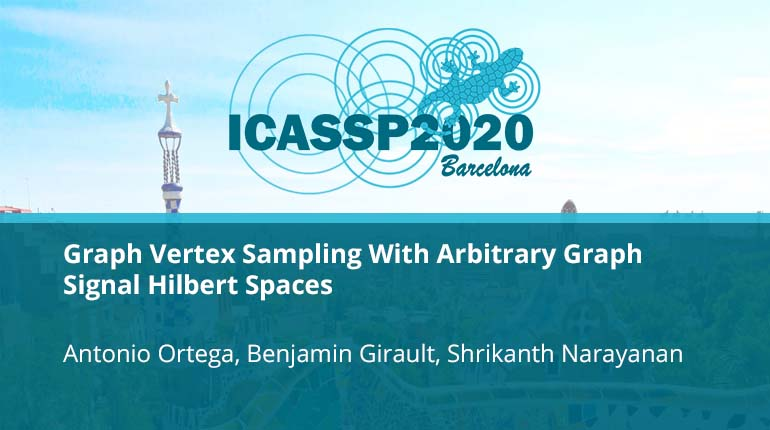Graph Vertex Sampling With Arbitrary Graph Signal Hilbert Spaces

Collection:Graph vertex sampling set selection aims at selecting a set of vertices of a graph such that the space of graph signals that can be reconstructed exactly from those samples alone is maximal. In this context, we propose to extend sampling set selection bas
• IEEE MemberUS \$11.00
• Society MemberUS \$0.00
• IEEE Student MemberUS \$11.00
• Non-IEEE MemberUS \$15.00
Purchase

Graph Vertex Sampling With Arbitrary Graph Signal Hilbert Spaces

Graph vertex sampling set selection aims at selecting a set of vertices of a graph such that the space of graph signals that can be reconstructed exactly from those samples alone is maximal. In this context, we propose to extend sampling set selection bas